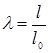# 6. Stress and deformation

When a force is applied to a body then that body will be deformed. This is true for any material, including polymers. The picture below shows a rod that is deformed by a compression force (left) and by a tensile force (right). The original not deformed rod is shown in the middle.A body subjected to a force will be deformed (commons.wikimedia.org).

We define the deformation or strain (e) as the change of length of the body (Dl) divided by the original length of the body (l0):Example: When a body with a length of 100 mm is stretched by 2 mm then the strain is 2 / 100 = 0.02.

Instead of the force (F) it is common to use stress instead (s), which is the force per unit area (A) in the body:Example: A force of 100 N on a body with a surface of 1000 cm2 (= 0.1 m2 ) will cause a stress of 100 / 0.1 = 1000 N/m2, which is the same as 1000 Pa.

In case of a small deformation the stress is proportional to the deformation. This fact is known as Hooke’s law. It says that the stress (s) is equal to the deformation (e) times the  elasticity modulus (E):The elasticity modulus is also called Young’s modulus . It is a material property.

Example: Steel has an elasticity modulus of 210,000 MPa (1 MPa = 106 N/m2). In order to create a strain of 0.02 in the bar a stress equal to 210,000 x 0.01 = 2,100 MPa is needed.

With this set of equations the stresses and strains in many situations are well described as long as the strain is small: not more than something like 0.03. When a body is deformed more than a few percent than Hooke’s law is not valid anymore.

Most polymers can be deformed much more than a few percent, especially when they are in the rubber phase. Think of a rubber band that can be stretched five to ten times before it breaks. Therefore, we need another way to describe the relation between stress and deformation in polymers.

It is common to specify the deformation of the polymer by the stretch ratio (l), which is the new length (l) divided by the original length (l0):Example: A body has an original length of 10 cm. If the body is stretched to a length of 15 cm then the stretch ratio is 15 / 10 = 1.5. If that same body would have been compressed to 5 cm then the stretch ratio is 5 / 10 = 0.5.

In case of large deformations, where Hooke’s law is not valid, the relation between stress and stretch ratio is as follows:In this equation G is the shear modulus of the body. Like the elasticity modulus it is a material property. Usually the shear modulus is about 1/3 of the elasticity modulus (G = E/3).

Example: Rubber has a shear modulus of about 10 MPa. If the rubber is stretched 2 times then the stress in the rubber will be 10 x (22 – ½) = 35 MPa. In case of undeformed rubber the stretch ratio is 1 and the stress will be 10 x (1 – 1) = 0 MPa.

## Summary:

• A stress will cause a deformation in a body.
• In case of small deformations the stress is proportional to the strain (Hooke’s law).
• Hooke’s law says that the stress is equal to the elasticity modulus times the strain.
• In case of large deformations the stress is better described by using the stretch ratio and the shear modulus.
• Both the shear modulus and the elasticity modulus are material properties. In a good approximation the shear modulus is 1/3 of the elasticity modulus.